# Python | Visualize graphs generated in NetworkX using Matplotlib

Prerequisites: Generating Graph using Network X, Matplotlib Intro
In this article, we will be discussing how to plot a graph generated by NetworkX in Python using Matplotlib. NetworkX is not a graph visualizing package but basic drawing with Matplotlib is included in the software package.

Step 1 : Import networkx and matplotlib.pyplot in the project file.

## Python3

 `# importing networkx ` `import` `networkx as nx`   `# importing matplotlib.pyplot` `import` `matplotlib.pyplot as plt`

Step 2 : Generate a graph using networkx.
Step 3 : Now use draw() function of networkx.drawing to draw the graph.
Step 4 : Use savefig(“filename.png”) function of matplotlib.pyplot to save the drawing of graph in filename.png file.

Below is the Python code:

## Python3

 `# importing networkx ` `import` `networkx as nx` `# importing matplotlib.pyplot` `import` `matplotlib.pyplot as plt`   `g ``=` `nx.Graph()`   `g.add_edge(``1``, ``2``)` `g.add_edge(``2``, ``3``)` `g.add_edge(``3``, ``4``)` `g.add_edge(``1``, ``4``)` `g.add_edge(``1``, ``5``)`   `nx.draw(g)` `plt.savefig(``"filename.png"``)`

Output: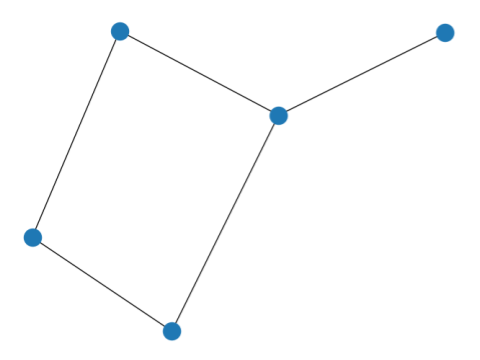To add numbering in the node add one argument with_labels=True in draw() function.

## Python3

 `# importing networkx ` `import` `networkx as nx` `# importing matplotlib.pyplot` `import` `matplotlib.pyplot as plt`   `g ``=` `nx.Graph()`   `g.add_edge(``1``, ``2``)` `g.add_edge(``2``, ``3``)` `g.add_edge(``3``, ``4``)` `g.add_edge(``1``, ``4``)` `g.add_edge(``1``, ``5``)`   `nx.draw(g, with_labels ``=` `True``)` `plt.savefig(``"filename.png"``)`

Output: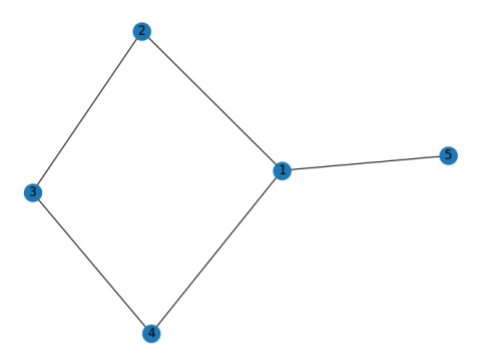Different graph types and plotting can be done using networkx drawing and matplotlib.

Note** : Here keywords is referred to optional keywords that we can mention use to format the graph plotting. Some of the general graph layouts are :

1. draw_circular(G, keywords) : This gives circular layout of the graph G.
2. draw_planar(G, keywords) :] This gives a planar layout of a planar networkx graph G.
3. draw_random(G, keywords) : This gives a random layout of the graph G.
4. draw_spectral(G, keywords) : This gives a spectral 2D layout of the graph G.
5. draw_spring(G, keywords) : This gives a spring layout of the graph G.
6. draw_shell(G, keywords) : This gives a shell layout of the graph G.

Example :

## Python3

 `# importing networkx` `import` `networkx as nx` `# importing matplotlib.pyplot` `import` `matplotlib.pyplot as plt`   `g ``=` `nx.Graph()`   `g.add_edge(``1``, ``2``)` `g.add_edge(``2``, ``3``)` `g.add_edge(``3``, ``4``)` `g.add_edge(``1``, ``4``)` `g.add_edge(``1``, ``5``)` `g.add_edge(``5``, ``6``)` `g.add_edge(``5``, ``7``)` `g.add_edge(``4``, ``8``)` `g.add_edge(``3``, ``8``)`   `# drawing in circular layout` `nx.draw_circular(g, with_labels ``=` `True``)` `plt.savefig(``"filename1.png"``)`   `# clearing the current plot` `plt.clf()`   `# drawing in planar layout` `nx.draw_planar(g, with_labels ``=` `True``)` `plt.savefig(``"filename2.png"``)`   `# clearing the current plot` `plt.clf()`   `# drawing in random layout` `nx.draw_random(g, with_labels ``=` `True``)` `plt.savefig(``"filename3.png"``)`   `# clearing the current plot` `plt.clf()`   `# drawing in spectral layout` `nx.draw_spectral(g, with_labels ``=` `True``)` `plt.savefig(``"filename4.png"``)`   `# clearing the current plot` `plt.clf()`   `# drawing in spring layout` `nx.draw_spring(g, with_labels ``=` `True``)` `plt.savefig(``"filename5.png"``)`   `# clearing the current plot` `plt.clf()`   `# drawing in shell layout` `nx.draw_shell(g, with_labels ``=` `True``)` `plt.savefig(``"filename6.png"``)`   `# clearing the current plot` `plt.clf()`

Outputs :
Circular Layout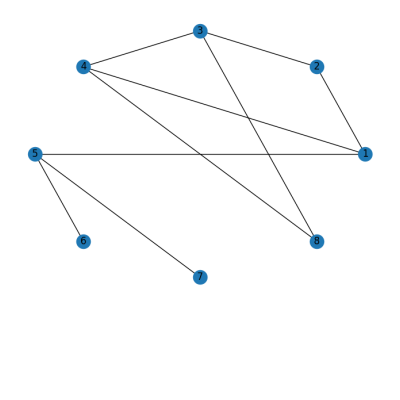Planar Layout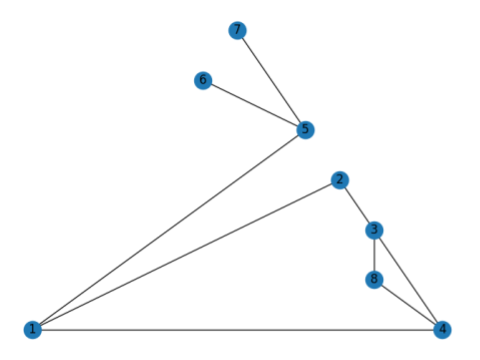Random Layout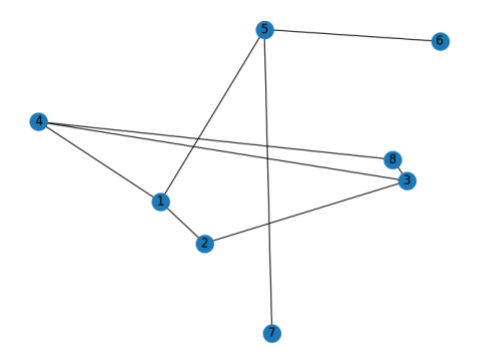Spectral Layout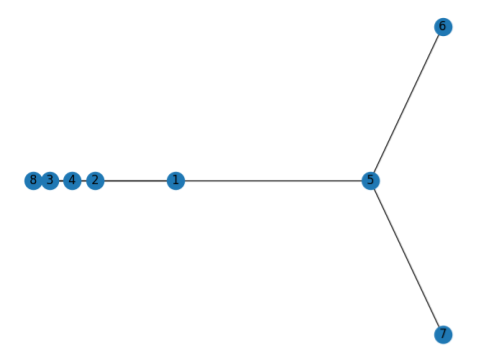Spring Layout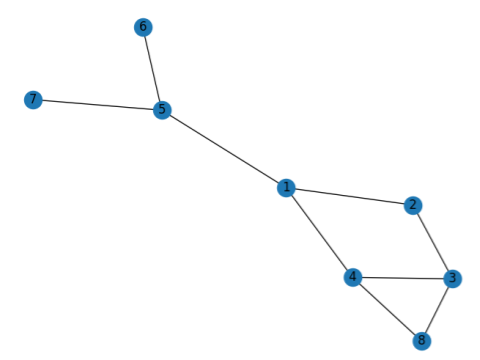Shell Layout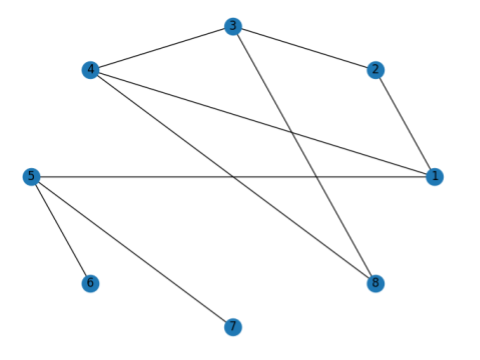Reference : NetworkX Drawing Documentation

Whether you're preparing for your first job interview or aiming to upskill in this ever-evolving tech landscape, GeeksforGeeks Courses are your key to success. We provide top-quality content at affordable prices, all geared towards accelerating your growth in a time-bound manner. Join the millions we've already empowered, and we're here to do the same for you. Don't miss out - check it out now!

Previous
Next